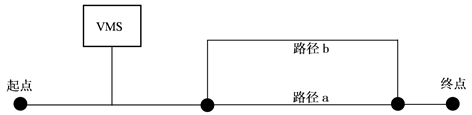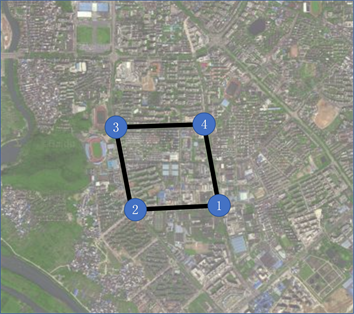﻿ 基于移动互联交通信息的城市交通诱导控制协同研究

# 基于移动互联交通信息的城市交通诱导控制协同研究 Research on Collaboration of Urban Traffic Guidance Control Based on Mobile Internet Traffic Information

Abstract: The paper conducts SP survey on the traveler’s path selection behavior under the mobile Internet traffic guidance information, uses SPSS software to screen and eliminates the influencing factors, and predicts the probability of the traveler changing the path by establishing a Logit regression model. Enlightenment from the collaborative control strategy of induced control is concluded. Taking the minimum sum of road network travel time and delay time as the objective function, a traffic guidance control collaborative model is established, and the collaborative model algorithm is optimized by genetic algorithm. A small road network with four intersections in Guilin is used as an example to analyze the model. Comparative analysis of the results before and after optimization shows that the model achieves a reduction in the total travel time of the road network, which verifies the feasibility of the model.

1. 引言

2. 出行者路径选择行为调查与分析

2.1. 出行者路径选择行为调查

2.1.1. SP调查问卷设计

(1) 影响因素调查

① 个人属性。包括：年龄、年收入、受教育水平、驾龄、对路网的熟悉程度等；

② 出行特性。包括出行目的、出行耗时、出行次数等；

③ 信息属性。包括对VMS的关注程度、对VMS信息准确度的评价、偏好的VMS发布信息的形式等；

④ 改变路径倾向。包括改变路径考虑的主要因素、何种情况下关注诱导信息等。

(2) 假设情景调查Figure 1. Hypothetical scenario diagram

2.1.2. 调查描述

2.2. 路径选择行为模型Table 1. Logistic regression parameter estimation results

$\begin{array}{c}p=\mathrm{exp}\left(1.751-0.144{x}_{1}+0.1379{x}_{2}+1.142{x}_{3}+1.492{x}_{4}+1.137{x}_{5}\\ \text{\hspace{0.17em}}\text{\hspace{0.17em}}+1.358{x}_{6}+1.183{x}_{7}\right)/\left[1+\mathrm{exp}\left(1.751-0.144{x}_{1}+0.1379{x}_{2}\\ \text{\hspace{0.17em}}\text{\hspace{0.17em}}+1.142{x}_{3}+1.492{x}_{4}+1.137{x}_{5}+1.358{x}_{6}+1.183{x}_{7}\right)\right]\end{array}$ (1)Table 2. Correspondence of independent variables

2.3. 模型分析

(1) 驾龄的影响

(2) VMS准确度的影响

(3) VMS关注程度的影响

(4) 改变路径选择因素的影响

2.4. 对制定交通诱导控制协同策略的启示

(1) 交通管理者通过VMS发布诱导信息时，应及时准确地更新诱导信息，提高出行者对VMS诱导信息的满意度和信任度，避免诱导信息与实际情况存在较大误差，导致出行者降低对VMS诱导信息的信任度，对交通诱导系统发挥诱导作用产生消极影响。

(2) 根据假设情景调查分析结果，VMS在不同情况下发布不同类型的诱导信息能够起到不同程度的诱导效果。因此，交通管理者通过VMS发布诱导信息时，可根据路段的实际行程距离、预测得到的行驶时间和其他路网情况，选择对出行者发布描述型诱导信息还是预测型诱导信息，以最大限度地提高出行者对诱导信息的依从性，达到最佳的诱导效果。

(3) 根据路径选择模型的分析结果，出行者对VMS交通诱导信息的关注程度越高，其依从诱导信息进行改变路径的概率越大，诱导效果越显著。因此，交通管理者可通过公众号、车载广播、政府平台和论坛等途径提高出行者对VMS的认知和关注，进而提高出行者对VMS诱导信息的接受度和使用率，提高VMS的诱导效果，改善路网车流分布的均衡性。

3. 交通控制与交通诱导协同模型

3.1. 路段行驶时间计算模型

${T}_{1}=\frac{L}{V}$ (2)

V——路段车辆的平均行驶速度；

L可通过现场测量得到。V的表达式为：

$v=\frac{{v}_{f}}{2}\left(1+\sqrt{1-\frac{4x}{{v}_{f}{k}_{j}}}\right)$ (3)

${T}_{1}=\frac{{k}_{j}{L}_{\alpha }\left(1-\sqrt{1-\frac{4x}{{v}_{f}{k}_{j}}}\right)}{2x}$ (4)

3.2. 交叉口延误模型

${T}_{2}=0.38C\frac{{\left(1-\lambda \right)}^{2}}{1-\lambda x}+173{x}^{2}\left[\left(x-1\right)+\sqrt{{\left(x-1\right)}^{2}+\frac{16x}{S}}\right]$ (5)

$\lambda$ ——绿信比；

X——饱和度；

S——信号交叉口饱和流量。

3.3. 协同模型的建立

$\begin{array}{l}f=\mathrm{min}\underset{\alpha =1}{\overset{n}{\sum }}\left({T}_{1}+{T}_{2}\right)\\ \text{s}\text{.t}\text{.}\text{\hspace{0.17em}}\left\{\begin{array}{l}\stackrel{¯}{x}=\frac{1}{n}\underset{1}{\overset{n}{\sum }}\text{ }\text{ }{x}_{i}<\psi ,\text{\hspace{0.17em}}\text{\hspace{0.17em}}i=1,2.3,\cdots ,n\\ {S}^{2}=\frac{1}{n}\underset{1}{\overset{n}{\sum }}{\left({x}_{i}-\stackrel{¯}{x}\right)}^{2}<\sigma ,\text{\hspace{0.17em}}\text{\hspace{0.17em}}i=1,2.3,\cdots ,n\\ \underset{\alpha \in A\left(k\right)}{\sum }{q}^{k}=O{D}_{k}^{n}+\underset{\alpha \in A\left(n\right)}{\sum }{u}^{n}\\ 0 (6)

3.4. 协同模型的遗传算法设计

$Fit\left(f\left(x\right)\right)=\mathrm{max}\left(M-f\left(x\right)\right)=\mathrm{max}\left(M-\mathrm{min}\underset{\alpha =1}{\overset{n}{\sum }}\left({T}_{\alpha 1}+{T}_{\alpha 2}\right)\right)$ (7)

$Fmin{f}^{\prime }\left(x\right)=f\left(x\right)+\sigma p\left(x\right)$ (8)

$p\left(x\right)={\sum }^{\text{​}}{\left(\mathrm{max}\left(0,-{g}_{i}\left(x\right)\right)\right)}^{2}+{\left({h}_{j}\left(x\right)\right)}^{2}$ (9)

${h}_{j}\left(x\right)$ ——约束条件中的等式约束。

4. 实例应用分析(1) 路网中的交叉口信号控制相位均为两相位；

(2) 交叉口信号周期为固定周期，交叉口1、交叉口4周期取60 s，交叉口2、交叉口3周期取80 s；

(3) 路网中道路的最大饱和度为0.4，最大饱和度方差为0.05；

(4) 路网中道路的车辆最大驶入率为1辆/s；

(5) 车流自由流行驶速度为22.3 m/s2，路段阻塞密度为0.131辆/m。

(6) 1-3、3-1、2-4、4-2的OD需求为0.4辆/s。

(7) 驶出率与流量的转化值为0.025。Table 3. Road network basic data sheetTable 4. Correspondence between parameter variables and x

5. 结语

 王川. 车路协同环境下交通控制与诱导协同研究[D]: [硕士学位论文]. 兰州: 兰州理工大学, 2014.

 高敏静. 城市交通控制与诱导系统协同优化研究[D]: [硕士学位论文]. 西安: 长安大学, 2011.

 李浩. 基于交通信息的城市网络路径诱导策略研究[D]: [硕士学位论文]. 合肥: 合肥工业大学, 2017.

 张卫华, 李梦凡. 不同交通信息诱导下驾驶员路径选择行为研究[J]. 重庆交通大学学报(自然科学版), 2018, 37(10): 86-93.

 史路. 诱导信息下出行者路径选择行为研究[D]: [硕士学位论文]. 成都: 西南交通大学, 2015.

 熊若曦. 基于车路协同的车辆诱导策略对路网交通流的影响研究[D]: [硕士学位论文]. 北京: 北京交通大学, 2016.

 刘权富. 城市交通控制与交通诱导协同研究[D]: [硕士学位论文]. 重庆: 重庆交通大学, 2014.

 杨波. 城市交通诱导与控制系统一体化集成理论与技术研究[D]: [硕士学位论文]. 桂林: 桂林电子科技大学, 2010.

Top